Ch 5. Rigid Body General Motion Multimedia Engineering Dynamics Fixed Axis Rotation Plane Motion Velocities Zero Velocity Point Plane Motion Accelerations Multiple Gears Rot. Coord. Velocities Rot. Coord. Acceleration
 Chapter - Particle - 1. General Motion 2. Force & Accel. 3. Energy 4. Momentum - Rigid Body - 5. General Motion 6. Force & Accel. 7. Energy 8. Momentum 9. 3-D Motion 10. Vibrations Appendix Basic Math Units Basic Equations Sections Search eBooks Dynamics Fluids Math Mechanics Statics Thermodynamics Author(s): Kurt Gramoll ©Kurt GramollDYNAMICS - CASE STUDY SOLUTION This problem involves two rotating disks, which suggests using two separate coordinate systems.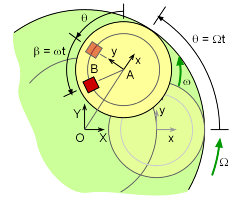Angles θ and β orientation The main disk's angular velocity Ω is a constant 1 rad/s about the fixed X-Y axes. The rider disk is attached to the main disk 3 meters from the center and has a constant angular velocity ω of 2 rad/s relative to the main disk. Both disks have no angular acceleration (dω/dt = 0, dΩ/dt = 0). The rotating x-y axes are attached to the main disk and rotate with the main disk. The rider is 1.5 m from the rider-disk center. The question requires the velocity at t = 2.4 s. Since both the time and angular velocities are known, the rotational position of both disks can be determined by θ = Ω t and β = ω t. The disks are assumed to be at full speed at t = 0.0 s. This ignores all start up accelerations. Since there are no angular accelerations, all tangential linear accelerations are zero. However, the normal linear acceleration are not zero. Use the relative velocity equation in the I-J fixed coordinate system (the i-j system could also be used),      aB = aA + dΩ/dt × rB/A + Ω × (Ω × rB/A)             + 2 Ω × vB/A + aB/A Analyze each term in the equation. Term aA (motion of A about center pt. O)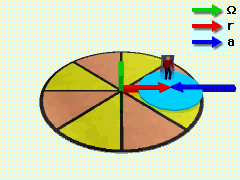Acceleration of A The first and simplest term is aA.      aA = dΩ/dt × rA/O + Ω × (Ω × rA/O)           = 0 + 1K × [1K × 3(cosθI + sinθJ)]           = 3(cos(Ωt)I - 3(sin(Ωt)J           = -3 sin(1(2.4))I - 3 cos(1(2.4))J           = -2.21 I - 2.03J m/s2 Notice that all the vectors can be described in the rotating i-j or the non-rotating I-J coordinate system. However, do not mix coordinate systems.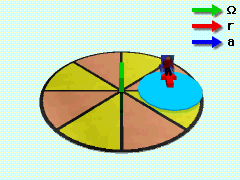Acceleration of B Due to Rotation Terms dΩ/dt×rB/A + Ω×(Ω×rB/A) Next, look at the rotating axis terms:      dΩ/dt × rB/A + Ω × (Ω × rB/A) = 0 +                 + 1K × [1K × 1.5(-sin(θ+β)I + cos(θ+β)J)]            = 1.5 sin(Ωt+ωt)I - 1.5 cos(Ωt+ωt)J            = 1.5 cos(3(2.4))I - 1.5 sin(3(2.4)) J            = 1.190I - 0.912J m/s2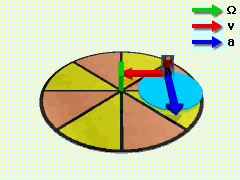Acceleration of B Due to Coriolis Effect Term 2Ω×vB/A (Coriolis acceleration) 2Ω × vB/A  =2Ω × (ω × rB/A)         = 2 (1K) × (2K × (-1.5 sin(θ+β)I + 1.5 cos(θ+β)J))         = 2K × (-3 sin(θ+β)J - 3 cos(θ+β)I)         = 6 sin(3t)I - 6 cos(3t)J         = 4.762I - 3.650J m/s2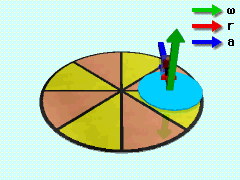Acceleration of B with Respect to A Term aB/A (motion of B with respect to A) aB/A = d Ω/dt × rB/A + ω × (ω × rB/A)        = 0 + 2K × 2K × [(-1.5 sin(θ+β)I                                        + 1.5 cos(θ+β)J)]        = 4 (1.5 sin(3t)I - 1.5cos(3t)J)          = 6 sin(3(2.4))I - 6 cos(3(2.4))J)]          = 4.762I - 3.650J  m/s2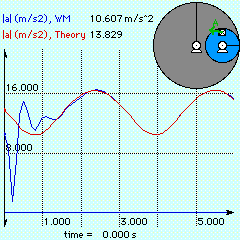Theory vs. Numerical Results All Terms Putting all terms together gives      aB = (2.21 + 1.19 + 4.76 + 4.76)I                   + (-2.03 - 0.912 - 3.65 - 3.65)J      aB = 12.92I - 10.24J m/s2 The total acceleration magnitude is 16.5 m/s2. Since a single g is 9.81 m/s2, the rider will experience 16.5/9.81 or 1.67 g's. This is a safe level of acceleration for a human.

Practice Homework and Test problems now available in the 'Eng Dynamics' mobile app
Includes over 400 problems with complete detailed solutions.
Available now at the Google Play Store and Apple App Store.